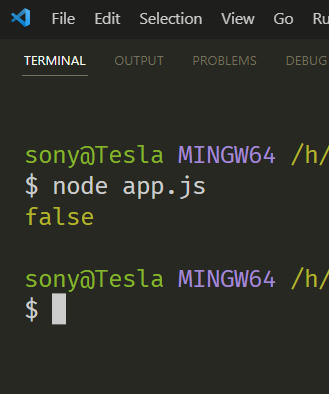# Javascript regular expression(regex) excercises confusion and clarity

``````/*
Excercise 02
1. First 5 can be only characters
2. Next can be any number of Non digits
3. Last 2 characters should be digit
*/

let sampleWord = "astronaut12"
let pwRegex = /(?=\w{5})(?=\D*d{2})/
console.log(pwRegex.test(sampleWord))
``````

Hello there, Where I am faltering I was expecting this to be true, but this is giving false.To find a digit use `\d`.

1 Like

Hello there @Archibald,

After the first five I have used this: `\D` and then `*` so that after the first five there may be characters(0 to infinite), but the last two should be numbers.

The total string is not restricted to only “7” (Only 5 characters + 2 numbers), but the constraint is first five are characters and the last two are numbers.

I think that this can also be reduced to this one → `let pwRegex = /(?=\w{5,})(?=d{2})/`

What Archibald is saying is you forgot the `\`. You told it “find any number of non-digit characters, and then the letter “d” twice.”

2 Likes

I got it. Thanks and sorry for the confusion.

You need to use ^ to match the beginning of input and need to use \$ to match the end of input.

The ‘Exercise 02’ implies that the first 5 can be any characters, not necesarily alphanumeric characters.

I don’t see that the look-ahead assertions (?=…) are required.

1 Like
``````/*
Excercise 02
1. First 5 can be only characters
2. Next can be any number of Non digits
3. Last 2 characters should be digit
*/

let sampleWord = "astronaut12"
let pwRegex = /(?=\w{5})(?=\D*\d{2})/
console.log(pwRegex.test(sampleWord))

//Simplyfying above regex
let pwRegex2 = /^\w{5,}\d{2}\$/
console.log(pwRegex2.test(sampleWord))
``````

You need to check for non digits.

This passes as ‘true’ but should be ‘false’: “Astron2aut12”

I fixed it like this:
`let pwRegex2 = /^\w{5}\D*\d{2}\$/`

If the first 5 can be any characters, not just alphanumeric (including underscore), then I suggest it should be:
/^.{5}\D*\d{2}\$/

1 Like